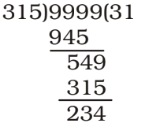## LCM and HCF

#### LCM and HCF

1.  L.C.M. of 2 , 4 , 5 is 3 9 6

1. As we know that ,

 LCM of fractions = LCM of numerators HCF of denominators

 LCM = LCM of 2, 4, 5 HCF of 3, 9, 6

##### Correct Option: B

As we know that ,

 LCM of fractions = LCM of numerators HCF of denominators

 LCM = LCM of 2, 4, 5 HCF of 3, 9, 6

 LCM = 20 3

1. Five bells begin to toll together and toll respectively at intervals of 6, 7, 8, 9 and 12 seconds. After how many seconds will they toll together again ?

1. Required time = LCM of 6, 7, 8, 9 and 12 seconds = 504 seconds

##### Correct Option: C

Required time = LCM of 6, 7, 8, 9 and 12 seconds = 504 seconds

1. The greatest number of four digits which when divided by 3, 5, 7, 9 leave remainders 1, 3, 5, 7 respectively is :

1. The difference between divisor and the corresponding remainder is equal.
LCM of 3, 5, 7 and 9 = 315
Largest 4-digit number = 9999∴ Number divisible by 315 = 9999 – 234 = 9765

##### Correct Option: A

The difference between divisor and the corresponding remainder is equal.
LCM of 3, 5, 7 and 9 = 315
Largest 4-digit number = 9999∴ Number divisible by 315 = 9999 – 234 = 9765
Required number = 9765 – 2 = 9763

1. Three bells ring simultaneously at 11a.m. They ring at regular intervals of 20 minutes, 30 minutes, 40 minutes respectively. The time when all the three ring together next is

1. LCM of 20, 30 and 40 minutes = 120 minutes
Hence, the bells will toll together again after 2 hours i.e. at 1 p.m.

##### Correct Option: B

LCM of 20, 30 and 40 minutes = 120 minutes
Hence, the bells will toll together again after 2 hours i.e. at 1 p.m.
Required time = 1 p.m.

1. Four bells ring at the intervals of 5, 6, 8 and 9 seconds. All the bells ring simultaneously at some time. They will again ring simultaneously after

1. The LCM of 5, 6, 8 and 9 = 360 seconds = 6 minutes

##### Correct Option: A

The LCM of 5, 6, 8 and 9 = 360 seconds = 6 minutes
Hence , They will again ring simultaneously after 6 minutes .